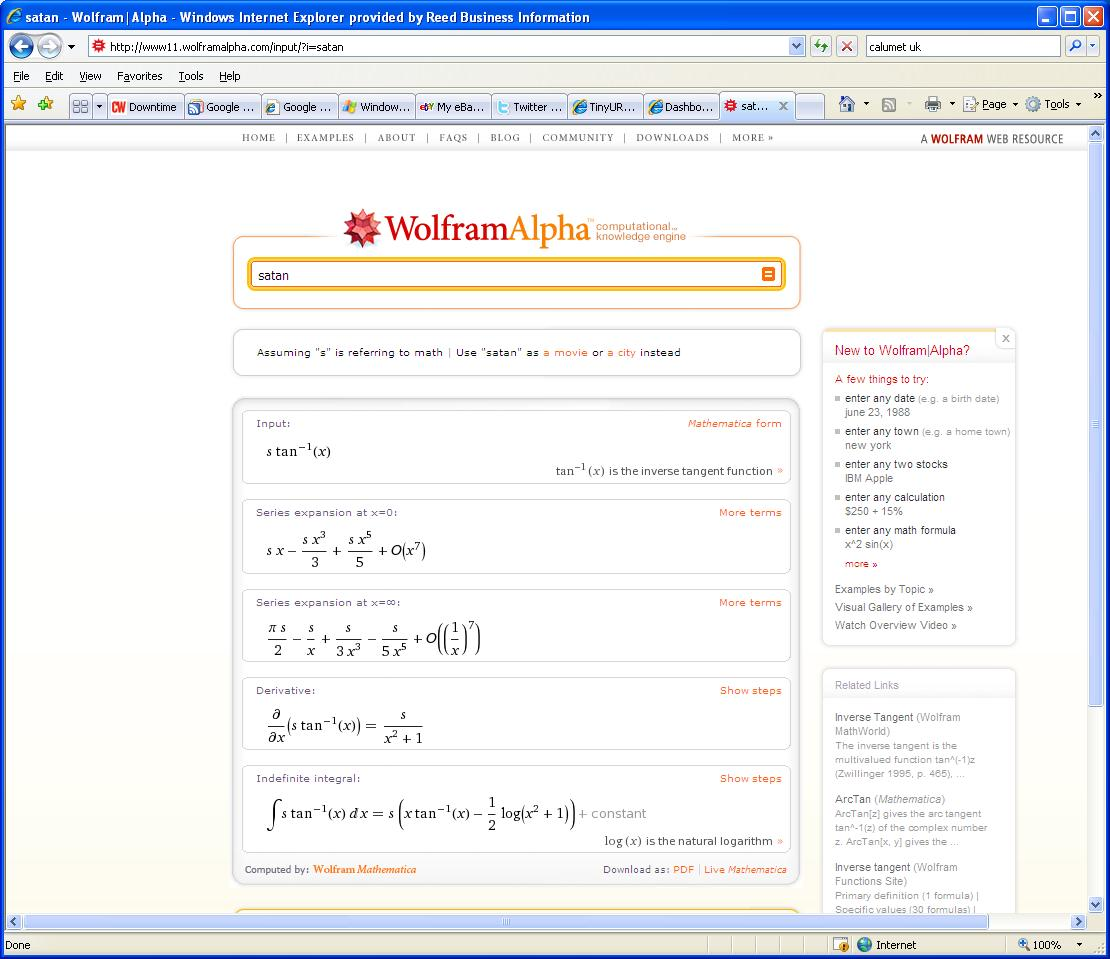# Wolfram Alpha calculates the Satan formula

Wolfram Alpha, the world’s latest search engine, uses clever maths to tell you things you don’t already know. In the name of research, Downtime asked Wolfram Alpha about Satan. Apparently Satan can be represented by a mathematical formula.Close